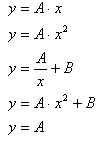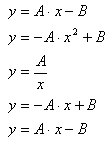EXTRA ACTIVITIES 3rd year of secondary education

 1. GRAPHS AND ALGEBRAIC EQUATIONS As with other previous windows, different graphs appear in the following window by changing the parameter “g”. 1.-Look carefully at all the different graphs that appear on the electronic board. Copy each graph into your exercise book. Then, choose the relevant algebraic equation from the following and write it next to each graph.2.- Each of the graphs above and their relevant algebraic equations correspond to a certain type of function. Find out what each one is called and write it down in your exercise book.

 2. A STRANGE GRAPH In this window there is a graph which is different to those you have seen so far, but could refer to a whole variety of everyday situations. Look at it carefully and complete the following activities in your exercise book. 3.- The graph represents prices charged in an airport car park according to the time that the car is parked there. Draw up a table of values that gives us the total amount charged for each period of time parked (work out the prices from one to twelve hours and remember that car parks always round up the time to the next full hour). Move the red point until you get the necessary information to complete the activity. 4.- Draw up a table of values that shows the amount charged for each hour parked from 1 to 10 hours. 5.- Write down five other everyday situations which could be represented with a graph like this one (i.e. one that gives the same shape rather than the exact same values).

3. A BIT OF PHYSICS

In this window there is a graphical representation of the change in potential and kinetic energy of a body falling through the Earth's gravity field (where air friction is zero and acceleration due to gravity is 10 metres a second squared for a mass of 1 Kg). Look carefully at the data given.

 6.- Draw up a table of values which shows: the values for potential and kinetic energy, speed and height at 1 second intervals from the point where the object starts to fall until it reaches the ground. 7.-What type of function corresponds to each of the graphs that appear? (Think back to activity 6.1 to remind you). 8.-Try and work out the exact algebraic equation that corresponds to each of the graphs in the window.Josep Mª Navarro CanutSpanish Ministry of Education. Year 2001